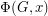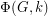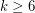# Half-integral flow polynomial values

 Importance: Medium ✭✭
 Author(s): Mohar, Bojan
 Subject: Graph Theory » Algebraic Graph Theory
 Keywords: nowhere-zero flow
 Posted by: mohar on: May 31st, 2007

Letbe the flow polynomial of a graph. So for every positive integer, the valueequals the number of nowhere-zero-flows in.

Conjecturefor every 2-edge-connected graph.

By Seymour's 6-flow theorem,for every 2-edge-connected graphand every integer.

It would be interesting to find any non-integer rational numberso thatfor every 2-edge-connected graph. It is known that zeros of flow polynomials are dense in the complex plane.

## Bibliography

* indicates original appearance(s) of problem.